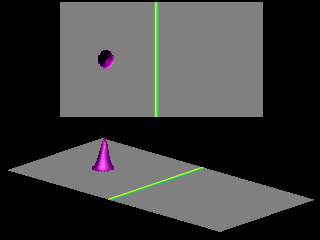﻿ Potential barrier
Quantum Mechanics
Tunneling: Potential barrier

Consider a classical particle (for example a ball) of mass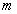with a kinetic energy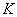moving toward a hill of height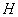. The ball rolls up the hill, transforming kinetic energy into potential energy given by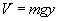, where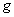is the acceleration due to gravitation. If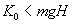the ball will not reach the top. At the point where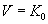the ball reverses its direction and rolls back from the slope. If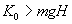the ball will roll over the top of the hill and will run down from the hill on the other side.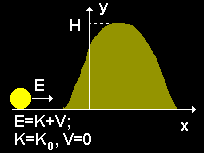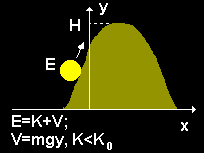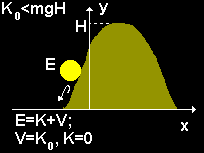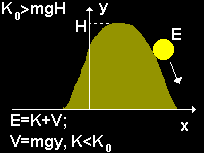Consider now a particle with energy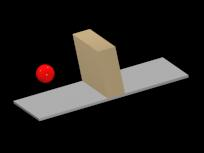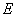approaching a potential step of height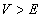. Classically the particle would be reflected by the step. A quantum particle incident from the left has a nonzero probability for being found to the right of the step. The animations show the tunneling of Gaussian wave packets through rectangular barriers of height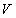and width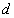(central strip). For visualization purposes, the probability of the transmitted wave (i.e. the wave appearing on the r.h.s. of the strip) has been rescaled.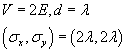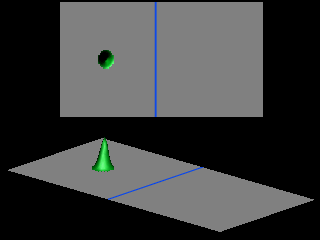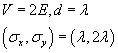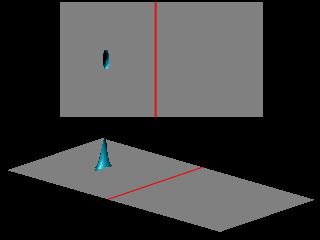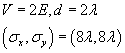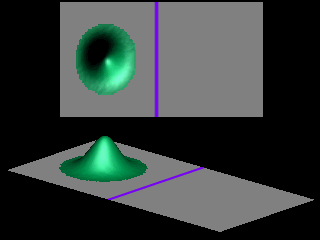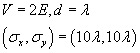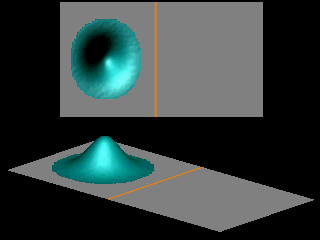The shape of the transmitted wave packet depends on the width of the initial wave packet.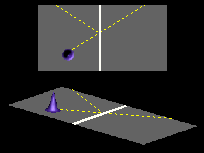The probability for tunneling vanishes exponentially with the thickness of the barrier. For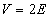and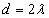the tunneling probability is less than 0.0000000001. For the potential barrier depicted at the right, the momentum of the particle in the direction parallel to the barrier is conserved. Therefore the tunneling process does not change the direction of the transmitted wave, as shown in the next animation.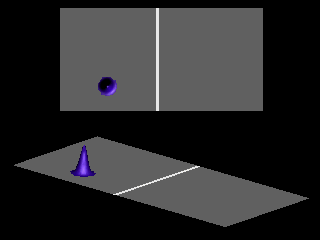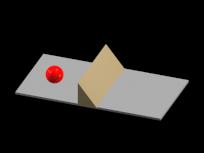Qualitatively the tunnel effect does not depend on the shape of the potential barrier, as illustrated in the next animation for the case of a triangular barrier.